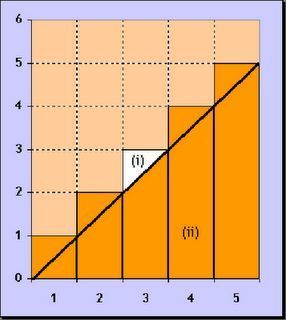## Friday, 6 February 2009

### SUMS INSIDE POWER SETLet's consider the set of integers less or equal than a given one:

$latex \displaystyle A=\{1,2,3,...,n\}\:\:n\in\mathbb{N}$

If we add all the elements in this set, we have:

$latex \displaystyle S=\sum_{i\in A}{i}=\sum_{i=1}^n{i}=\frac{n^2+n}{2}$

If we look, at the figure, we can see how the sum ,is equal to the area below the "ladder" plotted:

$latex \displaystyle S=S_{(i)}+S_{(ii)}$

Where $latex S_{(i)}$ is the area formed for $latex n$ small triangles, with a $latex \displaystyle \frac{1}{2}$ area, each one; And $latex S_{(ii)}$ is the area of an isosceles triangle with an equal base and height of $latex n$.

$latex \displaystyle S_{(i)}=\frac{1}{2}\cdot n;\quad S_{(ii)}=\frac{1}{2}\cdot n \cdot n;$

And if the aim, of this blog, where to be rigurous instead of, to give simple ideas, an induction proof, should fit here, perfectly .
To extend this result, to the sum of all the elements, in the subsets included, in the Power Set of integers less or equal to a given one:

$latex \displaystyle P(A) = \{X:X\subseteq A=\{1,2,3,...,n\}\}$

There are $latex 2^n$ subsets in $latex \displaystyle P(A)$, (see  and ):

$latex \displaystyle |P(A)| = 2^{|A|} =2^n$

If $latex \displaystyle i\in A$, then this element is in half of the subsets of $latex \displaystyle P(A)$ (observe the relation between the binary digits and the power set ): $latex \displaystyle 2^{n-1}$
To get the final result, it is only necessary to multiply both expresions :

$latex \displaystyle h(n)=\sum_{i\in X\subseteq P(A)}{i}= \frac{n^2+n}{2} \cdot 2^{n-1}=(n^2+n) \cdot 2^{n-2}$

NOTE:

$latex \displaystyle h(n)=A001788(n)$

$latex \displaystyle\lim_{x \to{+}\infty}{\frac{h(n+1)}{h(n)}}\displaystyle\lim_{x \to{+}\infty}{2+\frac{4}{x}}=2$
Archives:
References:

-A000217 The On-Line Encyclopedia of Integer Sequences!
-A001788 The On-Line Encyclopedia of Integer Sequences!
-Powerset Construction Algorithm Shriphani Palakodety.
-Course Notes 8: Size of the Power Set. Chris Nowlin.